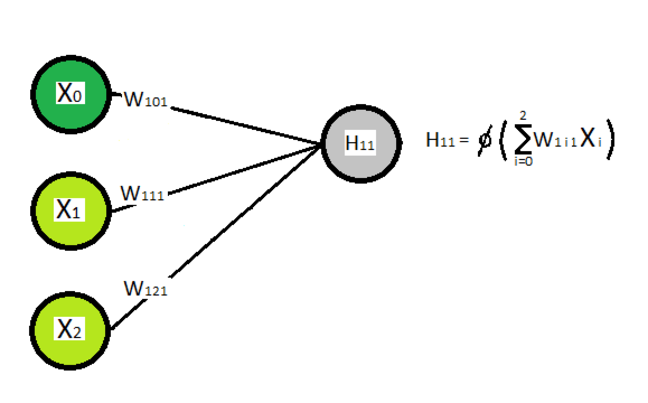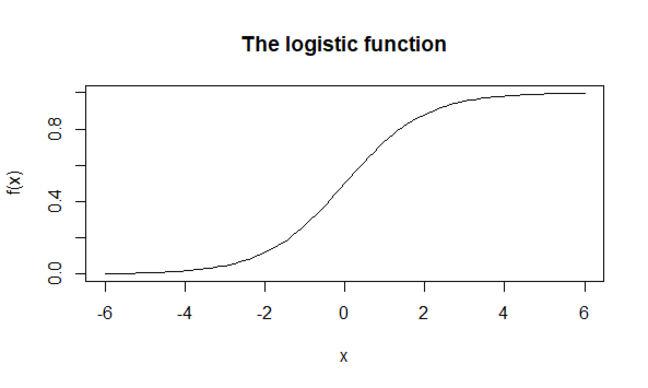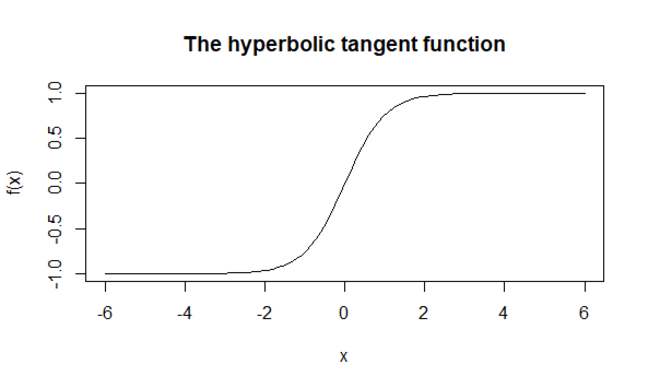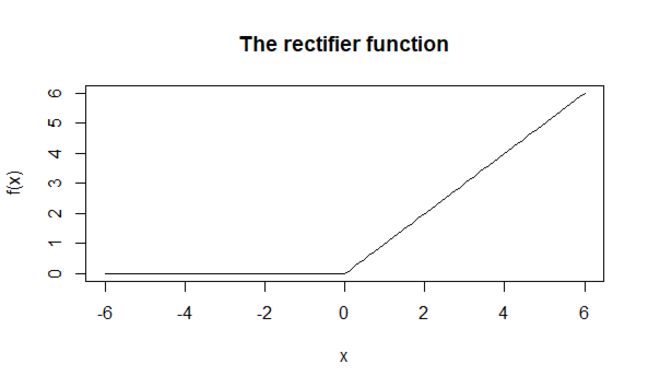## Want to keep learning?

This content is taken from the The Open University & Persontyle's online course, Advanced Machine Learning. Join the course to learn more.
2.18

# Activation Functions

As explained previously, hidden variables/nodes in a neural network are non-linear functions of the variables/nodes of the previous layer. In basic feed-forward neural networks they are typically some non-linear function, $$\phi$$ of the weighted sum of these variables in the previous layer.

Since we may have more than one hidden layer, we will refer to the jth hidden node in the ith hidden layer as $$H_{i,j}$$. With $$N_{i,j}$$ we will refer to both hidden nodes, where $$N_{i,j}=H_{i,j}$$ and, when $$i=0$$ the input features, where $$N_{0,j}=X_j$$ we can give a general equation for all hidden nodes in a network as:

$H_{i,j}=\phi (\sum_{k=0}^N w_{i,k,j} N_{i-1,j})$

Where we recall that the weights of the weighted sum being the edge weights on the edges leading into the hidden node.Given such a form, the question becomes what the non-linear function, $$\phi$$ should be. Typically the same non-linear function is chosen for all hidden nodes in a particular layer, but different layers may have different functions. We look at three common choices here.

### The Logistic Function

The logistic function squashes its argument (in this case the weighted sum) between 0 and 1. At $$x=0$$ the logistic function takes the value $$0.5$$, and it is also at this point that its gradiant is at the steepest.

$logistic(x)=\frac{e^x}{e^x+1}=\frac{1}{1+e^{-x}}$#### The sigmoid function?

The logistic function is sometimes referred to as the sigmoid function. This should be avoided, as a sigmoid function is simply.any function that possesses a S-like shape. As such both the logistic and the tanh function are examples of sigmoid functions.

### The Hyperbolic Tangent Function

The hyperbolic tangent, or tanh, function is similar to the logistic function, but squashes its argument between -1 and 1. At $$x=0$$ the tanh function takes the value $$0$$, and it is also at this point that its gradiant is at the steepest.

$tanh(x)=\frac{e^x-e^{-x}}{e^x+e^{-x}}$### The Rectifier Function

Both the logistic and hyperbolic tangent functions can face the problem that for most values of $$x$$ their gradient is very small. In deep neural networks (networks with many hidden layers) this can lead to ‘the vanishing gradient problem’, making them difficult to successfully train. In such networks it is common to use alternative activation functions, the most popular of which is the rectifier function. The rectifier simply returns its argument, unless its argument is less than 0 in which case it returns 0.

$rectifier(x)=max(0,x)$Nodes that use the rectifier function as their activiation function are often referred to as rectified linear units, or RELU.

There are many other activation functions in use, even in basic feed forward networks of the type we are focusing on in this course. You can view a number of these in the wikipedia activation function article.